# University of Washington, CSE 142

## Lab 7: Arrays

Except where otherwise noted, the contents of this document are Copyright 2013 Stuart Reges and Marty Stepp.

lab document created by Marty Stepp, Stuart Reges and Whitaker Brand

# Basic lab instructions

• Mouse over if you're not sure what they mean!
• Talk to your classmates for help.
• You may want to bring your textbook to future labs to look up syntax and examples.
• Stuck? Confused? Have a question? Ask a TA for help, or look at the book or past lecture slides.
• Complete as much of the lab as you can within the allotted time. You don't need to keep working on these exercises after you leave.
• Feel free to complete problems in any order.
• Make sure you've signed in on the sign-in sheet before you leave!

# Today's lab

Goals for today:

• use arrays for storing lists of data
• practice using `for` loops to traverse and process array data
• pass arrays as parameters and returns from methods

# Making arrays

An array is an object that can store many values of the same type. Making an array requires declaring how many values the array can hold, and what type of values the array will hold. The array type and capacity cannot change once the array has been made.

```// makes an integer array with 4 slots in it. Default: all values are 0.
int[] arrayName = new int; ```
```// another way to make an array: makes an integer array with 4 slots, all values being 0
int[] arrayName = {0, 0, 0, 0};```
```// arrays can be of any type!
// when object arrays are made, default value of each slot is null.
String[] arrayName = new String;```

# Exercise : Array declaration syntax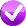Which of the following choices is the correct syntax for declaring/initializing an array of integers?

# Exercise : Quick initialization syntaxWhich of the following choices is the correct syntax for quickly declaring/initializing an array of integers to store a particular list of values?

# Accessing elements in an array

An array can be thought of as a series of cubbyholes. We can put values in and take values out of the cubbyholes by specifying which cubbyhole we want to work with. Just like with Strings, each cubby is indexed from 0 to the the length of the String/array.

```int[] a = new int;

// notice that unlike with Strings, we do not put a () after length
int length = a.length;
```
```int[] a = new int;

// makes the value at index 5 equal to 8.
// the pre-existing value at index 5 is ignored and overwritten.
a = 8;

// sets num equal to 8, the value at index 5 of a. Does not change the value of a.
int num = a;
```

# `ArrayIndexOutOfBoundsException`

If you try to access an index smaller than 0 or greater than `array.length - 1`, your program will crash with an `ArrayIndexOutOfBoundsException`.

```int[] a = new int;

// changes every value in array to equal 5.
for (int i = 0; i < a.length; i++) {
a[i] = 5;
}

// causes program to crash with ArrayIndexOutOfBoundsException
a = 5;

// also causes program to crash with ArrayIndexOutOfBoundsException
int num = a;```

# Exercise a: Array code tracingFill in the array with the values that would be stored after the code executes:

```int[] data = new int;
data = 3;
data = -18;
data = 5;
data = data;
```
index 0 1 2 3 4 5 6 7
value 3 3 0 0 5 0 0 -18

# Reference semantics

An array is an object.

Whether the elements of the array are objects or not depends on what type of values the array stores.

• If the array is storing (like `int`s, `double`s, or `char`s), then the elements are not objects.
• If the array stores objects (like `Scanner`s or `PrintStream`s), the array elements are objects.
continued on the next slide...

# Reference semantics

With primitive values, we use value semantics:
```int x = 5;
int y = x;
x = 10;
// here, x = 10, y = 5```
Setting `y = x` meant `y = ` the value that x had at that point in time. `y` does not change values when `x` changes values.

With Objects, we use reference semantics:
```int[] a = new int;     // a ==> [0, 0, 0, 0, 0]
int[] b = a;              // a ==> [0, 0, 0, 0, 0] <== b
a = 10;                // a ==> [10, 0, 0, 0, 0] <== b
b = 8;                 // a ==> [10, 8, 0, 0, 0] <== b```
Setting `b = a` means `b` and `a` now the same array. Changes to either `a` or `b` change both `a` and `b`.

# Exercise b: Array code tracingFill in the array with the values that would be stored after the code executes:

```int[] data = new int;
data = 3;
data = -18;
data = 5;
data = data;

int x = data;
data = 6;
data[x] = data * data;
```
index 0 1 2 3 4 5 6 7
value 3 3 0 0 6 9 0 -18

# Exercise : Array code tracing 2Fill in the array with the values that would be stored after the code executes:

```int[] list = {2, 18, 6, -4, 5, 1};
for (int i = 0; i < list.length; i++) {
list[i] = list[i] + (list[i] / list);
}
```
index 0 1 2 3 4 5
value 3 24 8 -5 6 1

# Exercise : PromptNumbers• Download the following file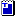PromptNumbers.java to your machine and open it with jGrasp.
• The program is supposed to prompt the user to enter several integers, store them into an array, then print those integers back out in forwards and backwards order. Finish the program so that it runs properly. Use an array to make your program flexible enough that it will work no matter how many integers the user wants to type.

# Arrays as parameter/return (declare)

```public static returnType methodName(arrayType[] parameterName) {   // pass array parameter
```
```public static returnArrayType[] methodName(parameters) {            // return array
```
• Arrays can be passed as parameters and returned from methods.
• This method takes an array of `double`s, and returns a new array of rounded `int`s:
```public static int[] roundAll(double[] realNums) {
int[] roundedNums = new int[realNums.length];
for (int i = 0; i < realNums.length; i++) {
roundedNums[i] = (int) Math.round(realNums[i]);
}
return roundedNums;
}
```

# Arrays as parameter/return (call)

• Below is an example usage of the `roundAll` method from the previous slide:
```import java.util.*; // to use Arrays

public class MyProgram {
public static void main(String[] args) {
double[] realNumbers = {5.5, 7.31, 8.09, -3.234234, 2.0, 0.0};
int[] roundedNumbers = roundAll(realNumbers);
System.out.println(Arrays.toString(roundedNumbers));
}
...
}

// Output: [5, 7, 8, -3, 2, 0]
```
Note: to print out the array we said `System.out.println(Arrays.toString(arrayName))`. Why's that? If we just say `System.out.println(arrayName)`, we'd get a weird jumble of letters and numbers. To get a well formatted array, we need to ask the `Arrays` class to turn the array into a nice String for us!

# `Arrays` class methods

In fact, the `Arrays` class has a ton of useful methods!
Method name Description
`Arrays.binarySearch(array, value)` returns index of value in a sorted array (< 0 if not found)
`Arrays.copyOf(array, length)` returns a new copy of an array
`Arrays.equals(array1, array2)` returns true if the two arrays contain same elements
`Arrays.fill(array, value)` sets every element to the given value
`Arrays.sort(array)` arranges the elements into sorted order
`Arrays.toString(array)` returns a string for the array, such as "[10, 30, -25, 17]"

# Exercise : array mysterySuppose that each array at right were passed as a parameter to the `mystery` method below. Fill in the boxes with the array contents after each method call.

Hint: it might be helpful to draw out an array an update its values as you trace through the code!
```public static void mystery(int[] a) {
for (int i = 0; i < a.length - 1; i++) {
if (a[i] < a[i + 1]) {
a[i] = a[i + 1];
}
}
}
```
 ```int[] a1 = {2, 4};mystery(a1);``` `{4, 4}` `[^0-9,]+` ```int[] a2 = {1, 3, 6};mystery(a2);``` `{3, 6, 6}` `[^0-9,]+` ```int[] a3 = {7, 2, 8, 4};mystery(a3);``` `{7, 8, 8, 4}` `[^0-9,]+` ```int[] a4 = {5, 2, 7, 2, 4};mystery(a4);``` `{5, 7, 7, 4, 4}` `[^0-9,]+` ```int[] a5 = {2, 4, 6, 3, 7, 9};mystery(a5);``` `{4, 6, 6, 7, 9, 9}` `[^0-9,]+`

# Exercise : PromptNumbers2

• Modify your `PromptNumbers.java` program from a previous exercise.
• Add a method that accepts an array as a parameter and prints the elements of that array in forward order.
• Then add a second method that accepts an array as a parameter and prints the elements of that array in backward order.

```    public static void main(String[] args) {
int count = console.nextInt();
int[] nums = new int[count];
...
printForward(nums);
printBackward(nums);
}

public static void printForward(int[] a) {
for (int i = 0; i < a.length; i++) {
System.out.println(a[i]);
}
}

public static void printBackward(int[] a) {
for (int i = a.length - 1; i >= 0; i--) {
System.out.println(a[i]);
}
}```

# Do we need to return arrays?

It depends!

• If we are modifying an array passed in as a parameter, we do not need to return the array. If we create `int[] a` in `methodX()` and then pass `a` into `methodY()`, any changes made to the parameter array in `methodY()` will also be reflected in `methodX()`.
• If `methodX()` calls `methodY()`, and then we make `int[] a` in methodY(), `a` must be returned to `methodX()` for `methodX()` to be able to use it.

# Exercise : do we need to return?

For the following, do we need to return the array? Write `yes` or `no`:
```// takes in an integer array, adds 5 to each element
public static ??? addFive(int[] a) {
for (int i = 0; i < a.length; i++) {
a[i] = a[i] + 5;
}
return a? no
}```
```// takes in an integer array, returns an array with each element increased by 5.
// does not modify given array.
public static ??? addFive(int[] a) {
int[] returnArray = new int[a.length];
for (int i = 0; i < a.length; i++) {
returnArray[i] = a[i] + 5;
}
return returnArray? yes
}```

# Exercise : Array reference mysteryWhat values are stored in the array at the comment in `main`? Note that the `incrementAll` method returns `void`, but does take an `int[]` parameter.

```public static void main(String[] args) {
int[] nums = {2, 4, -1, 3};
incrementAll(nums);

// HERE!
}

public static void incrementAll(int[] data) {
for (int i = 0; i < data.length; i++) {
data[i]++;
}
}
```
index 0 1 2 3
value 3 5 0 4

# Checkpoint: Congratulations!

Nice job making it this far--labs are tough! Feel free to work with the person next to you for the remaining slides. Labs are a unique opportunity (unlike homework) to collaborate directly on ideas, and practice peer programming.

These next problems get a little more challenging as we explore earlier concepts further.

We put a lot of problems in here so that you have plenty to refer back to later when working on homework. Don't feel bad if you don't finish all of them--Brett can't finish them all in a 50 minute lab, either! :)Forest the cat says good job!

# Exercise : max errors``` 1 2 3 4 5 6 7 8 9 10 ``` ```// Returns the largest value in the given array. public static int max(int data) { int max = 0; for (int i = 0; i < data[].length(); i++) { if (array[i] > max) { max = array[i]; } } return max[]; } ```

The above attempted solution to Practice-It problem "`max`" has some problems. Open Practice-It from the link above, copy/paste this code into it, and fix the errors. Complete the code so that it passes the test cases.

# Exercise - solution

 ``` 1 2 3 4 5 6 7 8 9 10 11 12 ``` ```// Returns the largest value in the given array. public static int max(int[] data) { // every value of data could be negative, in which case setting max = 0 is incorrect // max = data is safe as the value at the 0th index is definitely in data int max = data; for (int i = 1; i < data.length; i++) { if (data[i] > max) { max = data[i]; } } return max; } ```

# Exercise : jGRASP Debugger

• Download the fileTriangleCount.java to your machine and open it with jGrasp.
• This program explores patterns of digits for what are called the triangle numbers. The program counts how many times a triangle number ends in a particular 2-digit sequence. For example, the 15th triangle number is 120, which ends in the 2-digit sequence 20.
• Run the program and you will see a pattern: Most counts are 0 (not printed) and most of the rest are exactly 200. Which sequences have a count of 500?  3 28 53 78
• continued on the next slide...

# Exercise - jGRASP Debugger

• The program prints a progress dot every 200 iterations. Set a breakpoint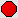on the `System.out.print` inside the `if` on line 23 and debug the program.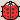• Once the program pauses, click and drag the array at left named `count`, and drop it on the main code window. This will show a view of its contents.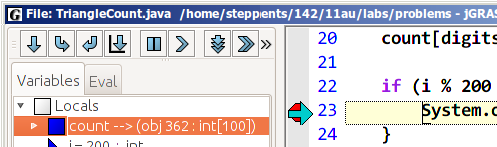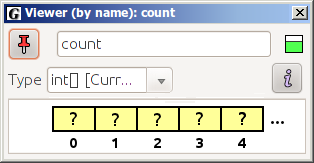• What are the first eight element values stored in the `count` array?
index 0 1 2 3 4 5 6 7
value 4 4 0 10 0 4 4 0

continued on the next slide...

# Exercise - jGRASP Debugger

• Leave the array viewer open and click the Resume button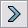. (This runs until the second time the `System.out.print` is about to execute, then stops.)
• What values do the first 8 array elements have now?
index 0 1 2 3 4 5 6 7
value 8 8 0 20 0 8 8 0
• This is a very long array with 100 total values.
Scroll to the far right in the array viewer. What values do the last 8 elements have?
index 92 93 94 95 96 97 98 99
value 0 0 0 8 8 0 0 0

# Exercise : `percentEven`Write a method named `percentEven` that accepts an array of integers as a parameter and returns the percentage of even numbers in the array as a real number. For example, if a variable named `nums` refers to an array of the elements `{6, 2, 9, 11, 3}`, then the call of `percentEven(nums)` should return `40.0`. If the array contains no even elements or no elements at all, return `0.0`.

Click on the check-mark above to try out your solution in Practice-it!

# Exercise : `minGap`Write a method named `minGap` that accepts an integer array as a parameter and returns the minimum 'gap' between adjacent values in the array. The gap between two adjacent values in a array is defined as the second value minus the first value. For example, suppose a variable called `array` is an array of integers that stores the following sequence of values:

```int[] array = {1, 3, 6, 7, 12};
```

The first gap is 2 (3 - 1), the second gap is 3 (6 - 3), the third gap is 1 (7 - 6) and the fourth gap is 5 (12 - 7). Thus, the call of `minGap(array)` should return `1` because that is the smallest gap in the array. If you are passed an array with fewer than 2 elements, you should return `0`.

Click on the check-mark above to try out your solution in Practice-it!

# Exercise : `swapAll`Write a method named `swapAll` that accepts two arrays of integers as parameters and swaps their entire contents. You may assume that the arrays passed are not null and are the same length.

For example, if the following arrays are passed:

```int[] a1 = {11, 42, -5, 27, 0, 89};
int[] a2 = {10, 20, 30, 40, 50, 60};
swapAll(a1, a2);
```

After the call, the arrays should store the following elements:

```a1: {10, 20, 30, 40, 50, 60}
a2: {11, 42, -5, 27, 0, 89}
```

# Exercise : `stretch`Write a method named `stretch` that accepts an array of integers as a parameter and returns a new array twice as large as the original, replacing every integer from the original array with a pair of integers, each half the original. If a number in the original array is odd, then the first number in the new pair should be one higher than the second so that the sum equals the original number.

For example, if a variable named `list` refers to an array storing the values `{18, 7, 4, 24, 11}`, the call of `stretch(list)` should return a new array containing `{9, 9, 4, 3, 2, 2, 12, 12, 6, 5}`. (The number 18 is stretched into the pair 9, 9, the number 7 is stretched into 4, 3, the number 4 is stretched into 2, 2, the number 24 is stretched into 12, 12 and the number 11 is stretched into 6, 5.)

Click on the check-mark above to try out your solution in Practice-it!

# Exercise : `equals`Write a method named `equals` that accepts two arrays of integers as parameters and returns `true` if they contain exactly the same elements in the same order, and `false` otherwise. Note that the arrays might not be the same length; if the lengths differ, return `false`. Do not call `Arrays.equals` in your solution.

For example, if the following arrays are declared:

```int[] a1 = {10, 20, 30, 40, 50, 60};
int[] a2 = {10, 20, 30, 40, 50, 60};
int[] a3 = {20, 3, 50, 10, 68};
```

The call `equals(a1, a2)` returns `true` but the call `equals(a1, a3)` returns `false`.

# If you finish them all...

If you finish all the exercises, try out our Practice-It web tool. It lets you solve Java problems from our Building Java Programs textbook.

You can view an exercise, type a solution, and submit it to see if you have solved it correctly.

Choose some problems from the book and try to solve them!